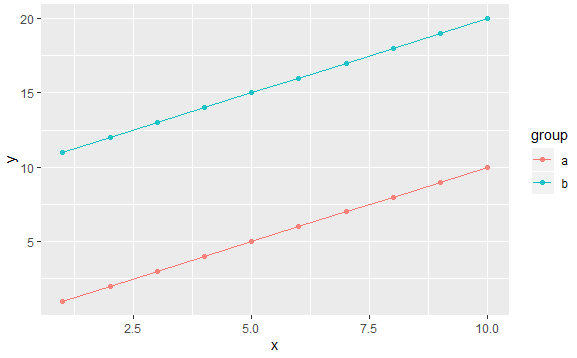2 views

I have a simple data frame that I'm trying to do a combined line and point plot using ggplot2. Supposing my data looks like this:

df <- data.frame(x=rep(1:10,2), y=c(1:10,11:20),

group=c(rep("a",10),rep("b",10)))

And I'm trying to make a plot:

g <- ggplot(df, aes(x=x, y=y, group=group))

g <- g + geom_line(aes(colour=group))

g <- g + geom_point(aes(colour=group, alpha = .8))

g

The result looks fine with one exception. It has an extra legend showing the alpha for my geom_point layer.How can I keep the legend showing group colors, but not the one that shows my alpha settings?

by

To remove the extra legend from your plot, you need to place the alpha argument outside the aesthetic function since aesthetic defined within aes(...) is mapped from the data, and a legend is created. And it appears that you wish to set alpha = 0.8 and map colour = group.i.e.,

df <- data.frame(x=rep(1:10,2), y=c(1:10,11:20),

group=c(rep("a",10),rep("b",10)))

g <- ggplot(df, aes(x = x, y = y, group = group))

g <- g + geom_line(aes(colour = group))

g <- g + geom_point(aes(colour = group), alpha = 0.8)

g

Output: